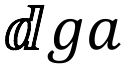# dga Release 0.1.6

A simple discrete genetic algorithm.

MIT
Install
``` pip install dga==0.1.6 ```

### DocumentationA simple discrete genetic algorithm for Python.

``````\$ pip install dga
``````

To get the git version do

``````\$ git clone https://github.com/msparapa/dga
``````

## Documentation and usage

This is a simple genetic algorithm where every design variable is modeled as a discrete bit array. Each bit can take a value of either `0` or `1`. Eg. A problem with a single parameter and 3 bits has the bit-string `000` corresponding to the lowest possible state.

To start, an initial population is generated with possible solutions randomly assigned. The cost function is evaluated for every possible solution. The lowest `50%` of evaluated solutions are kept, and the highest `50%` are removed. Children are then created from the top 50% by mixing the bit-strings of two parents. For example, the children of

``````Parent 1: 001
Parent 2: 010
``````

may look like

``````Child 1: 000
Child 2: 011
``````

There is a small chance that a bit may flip when creating the children, called mutation. The process runs again with the new population. The algorithm converges once `90%` of all bit-strings in the population are the same or the max generation limit has been reached.